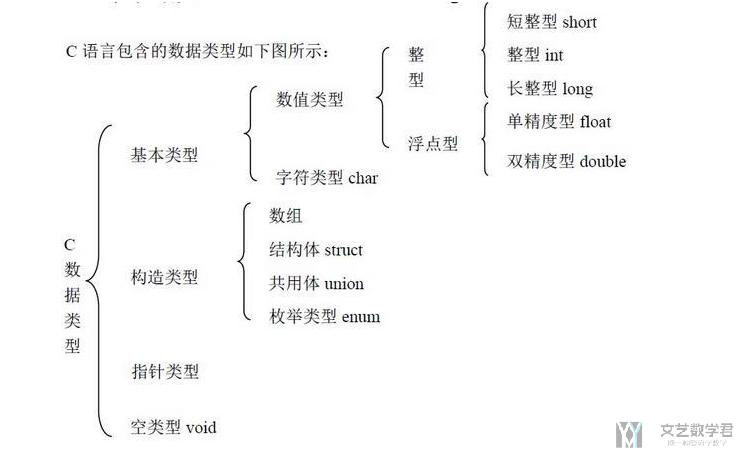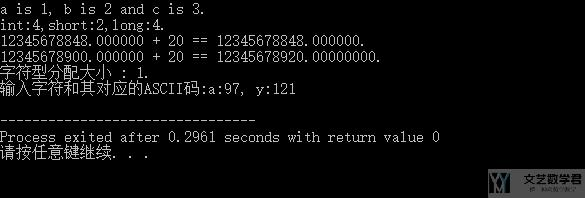# C学习笔记 – 数据类型

• A+

## 本文的主要内容• 数值类型（整型 : int，浮点型 : float）
• 字符类型 : char

### 源代码

1. #include<stdio.h>
2. /*
3.     关于数据结构的一些介绍
4.     这一部分介绍:常见的几种数据类型,包括int,float,char
5. */
6. int main(){
7.     /*
8.     介绍整型 : int,short,long
9.     */
10.     int a,b,c;
11.     a = 1;
12.     b = 2;
13.     c = a + b;
14.     printf("a is %d, b is %d and c is %d.\n",a,b,c); // 格式输出函数 printf
15.     // >> a is 1, b is 2 and c is 3.
16.     /*
17.     除了int外,还有short和long
18.     每种数据类型系统分配的大小不同
19.     int为4字节,short为2字节,long为8字节
20.     */
21.     printf("int:%d,short:%d,long:%d.\n",sizeof(int),sizeof(short),sizeof(long));
22.     // >> int:4,short:2,long:4
23.     // -----------------------------
24.     /*
25.     介绍浮点型 : float,double,long double
26.     */
27.     // float有效位数7, double有效位数16, long double有效位数19
28.     // 下面看一下数值溢出的情况
29.     float d_float = 123456.789e5;
30.     float f_float = d_float + 20;
31.     printf("%f + 20 == %f.\n",d_float,f_float);
32.     // >>12345678848.000000 + 20 == 12345678848.000000.=> 这个计算结果是错误的
33.     // 使用double类型可以解决这个问题
34.     double d_double = 123456.789e5;
35.     double f_double = d_double + 20;
36.     printf("%f + 20 == %0.8f.\n",d_double,f_double);
37.     // >> 12345678900.000000 + 20 == 12345678920.00000000. => 这个计算结果是正确的,同时%0.8f表示控制输出位数
38.     // ------------------------------
39.     /*
40.     介绍字符型 : char (分配一个字节)
41.     */
42.     char g_char='a',h_char=121; // 可以直接用''进行定义,也可以使用ASCII的值进行定义
43.     printf("字符型分配大小 : %d. \n", sizeof(g_char));
44.     // >> 字符型分配大小 : 1.
45.     printf("输入字符和其对应的ASCII码:%c:%d, %c:%d\n",g_char,g_char,h_char,h_char);
46.     // >> 输入字符和其对应的ASCII码:a:97, y:121
47.     return 0;
48. }• 微信公众号
• 关注微信公众号
•• QQ群
• 我们的QQ群号
•